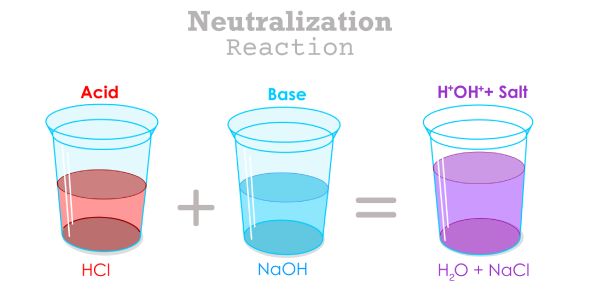# Neutralization Reaction Quiz Questions And Answers

Approved & Edited by ProProfs Editorial Team
At ProProfs Quizzes, our dedicated in-house team of experts takes pride in their work. With a sharp eye for detail, they meticulously review each quiz. This ensures that every quiz, taken by over 100 million users, meets our standards of accuracy, clarity, and engagement.
| Written by Aymanali
A
Aymanali
Community Contributor
Quizzes Created: 1 | Total Attempts: 365
Questions: 10 | Attempts: 367SettingsWelcome to the Neutralization Reaction Quiz! This quiz is designed to test your understanding of neutralization reactions, a fundamental concept in chemistry. You'll be challenged with questions about the reactions between acids and bases, the formation of salt and water, and the principles that govern these reactions.

Whether you're a student looking to reinforce your knowledge or someone curious about the fascinating world of chemistry, this Neutralization Reaction Quiz offers an engaging way to explore the topic. Dive in and see how well you understand the balance and transformation that occur in neutralization reactions. Good luck!

• 1.

### What is the product of a neutralization reaction between an acid and a base?

• A.

Salt

• B.

Water

• C.

Both Salt and Water

• D.

Neither Salt nor Water

C. Both Salt and Water
Explanation
In a neutralization reaction, the acid will produce H+ ions that react to neutralize the OH- ions produced by the base, forming neutral water. The other product will be an ionic salt.

Rate this question:

• 2.

### Which acid and base combination will produce NaCl and H2O?

• A.

HCl + NaOH

• B.

H2SO4 + NaOH

• C.

HNO3 + KOH

• D.

CH3COOH + KOH

A. HCl + NaOH
Explanation
Positive hydrogen ions from HCl and negative hydroxide ions from NaOH combine to form water. Positive sodium ions from NaOH and negative chloride ions from HCL combine to form the salt sodium chloride (NaCl), commonly called table salt.

Rate this question:

• 3.

### What is the pH of a neutral solution?

• A.

1

• B.

7

• C.

14

• D.

0

B. 7
Explanation
Ions are just atoms that have an electric charge on them, so H+ is a hydrogen atom with charge of 1. Even in pure water ions tend to form due to random processes (producing some H+ and OH- ions). The amount of H+ that is made in pure water is about equal to a pH of 7. That's why 7 is neutral.

Rate this question:

• 4.

### In a neutralization reaction, a strong acid and strong base form what type of solution?

• A.

Acidic

• B.

Basic

• C.

Neutral

• D.

None of the above

C. Neutral
Explanation
Neutral solution is obtained from this because acidic and basic solutions of same strength, nullify the effect of each other and the resulting solution has neither basic or acidic behavior. When a strong acid reacts with water, it makes only the solution dilute. It does not nullify or suppress the acidic behavior.

Rate this question:

• 5.

### What is the name of the reaction where an acid reacts with a metal oxide?

• A.

Combustion

• B.

Neutralization

• C.

Substitution

• D.

Reduction

B. Neutralization
Explanation
Metal oxides neutralise the effect of acids and release water, similar to the reaction of a base with an acid. Q. Since the products of reactions of acid + base and acid + metal oxide are same.

Rate this question:

• 6.

### Which indicator changes color at a pH of 7?

• A.

Phenolphthalein

• B.

Methyl orange

• C.

Bromothymol blue

• D.

Litmus

C. Bromothymol blue
Explanation
It's used to watch changes in pH; photosynthesis, where carbon dioxide is used up; and respiration (carbon dioxide is produced). This works because bromothymol blue can be deprotonated above a pH of 7.1, which creates a blue color, while the protonated form, below a pH of 7.1, is a yellow compound.

Rate this question:

• 7.

### What type of reaction is H2SO4 + 2NaOH → Na2SO4 + 2H2O?

• A.

Combustion

• B.

Neutralization

• C.

Substitution

• D.

Reduction

B. Neutralization
Explanation
The given equation, H2SO4 + 2NaOH → Na2SO4 + 2H2O, is a combination of a neutralization reaction and a double displacement reaction. It is a neutralization reaction because H2SO4 and NaOH neutralize each other, forming water and a salt (Na2SO4).

Rate this question:

• 8.

### What is formed when a base reacts with a non-metal oxide?

• A.

Salt

• B.

Water

• C.

Acid

• D.

Both Salt and Water

C. Acid
Explanation
When carbon dioxide is dissolved in water it produces carbonic acid. Therefore, when a base reacts with non-metal oxide both neutralize each other resulting production of salt and water.

Rate this question:

• 9.

### In the reaction between acetic acid and sodium hydroxide, what is the salt formed?

• A.

Sodium acetate

• B.

Sodium chloride

• C.

Sodium sulfate

• D.

Sodium nitrate

A. Sodium acetate
Explanation
The salt (Na) from the sodium acetate is changed with the hydroxide (OH) from the salt hydroxide (NaOH). This leaves the acetate ion, which mixes with the salt (Na) from the salt hydroxide to create sodium acetate.

Rate this question:

• 10.

### What is the term for the amount of acid that exactly neutralizes a given amount of base?

• A.

Equivalence point

• B.

Endpoint

• C.

Saturation point

• D.

None of the aboveBack to top